# Understanding the PV Diagram of a Monatomic Gas

• PhizKid
In summary, the gas in a PV diagram experiences three processes:1) constant pressure - Q = W2) cycle - W = Q + ΔU3) constant volume - ΔU = Qf

## Homework Statement

Consider the following PV diagram of one mole of a monatomic gas: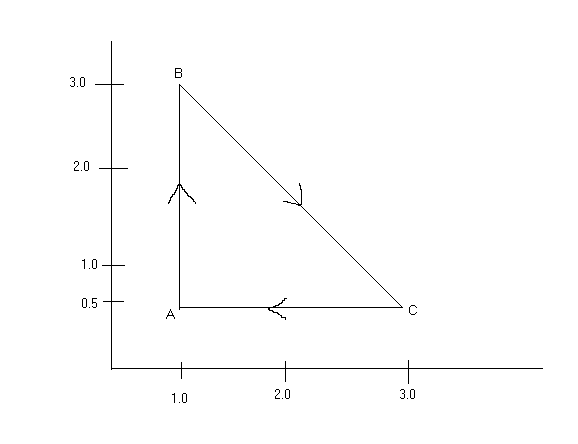The units are in atm and litres.

Find the change in internal energy, heat, and work for all 3 processes and for the cycle as well.

## Homework Equations

PV = nRT
ΔU = Q - W
ΔU = (3/2)*R*ΔT
Q = (5/2)*R*ΔT (for constant pressure)

## The Attempt at a Solution

For the work, I just found the areas:

A -> B: 0 J
B -> C: +3.5 J
C -> A: -1 J

For the cycle, the work done is just the total work added:
3.5 - 1 = 2.5 J

For the cycle, the Q is the same as the Work:
2.5 J

For the cycle, the ΔU = 0.

So far I have this (unless I did something wrong):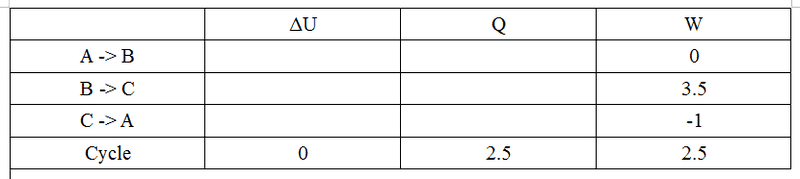Since for a constant volume process the ΔU = Q, I decided to do that A -> B first.

T_A = (0.5 atm)(1 L) = (1)(0.0821)T, T = 6.09 K
T_B = (0.5 atm)(1 L) = (1)(0.0821)T, T = 36.5 K

ΔT = 30.41 K

ΔU = (3/2)*R*ΔT = (3/2)*0.0821*30.41 = 3.75 J = Q

So: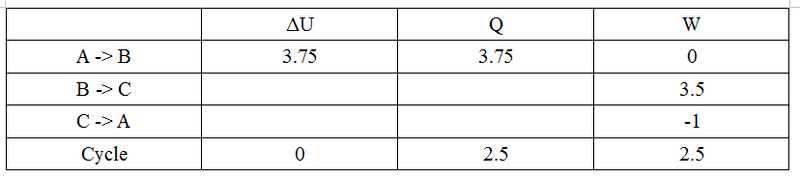The constant pressure process looks easier so I'll do C -> A next:

T_C = (0.5 atm)(3 L) = (1)(0.0821)T, T = 18.27 K
T_A = (0.5 atm)(1 L) = (1)(0.0821)T, T = 6.09 K

ΔT = -12.18

Q = (5/2)*R*ΔT = (5/2)*0.0821*(-12.18) = -2.5 J

ΔU = Q - W = (-2.5) - (-1) = -1.5

So: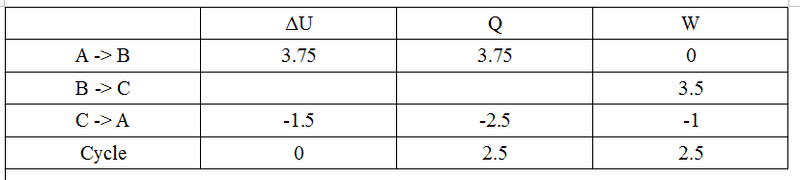Now I can just add up the unknown columns:

ΔU for B -> C: 3.75 + (-1.5) + x = 0, x = -2.25

Q for B -> C: 3.75 + (-2.5) + x = 2.5, x = 1.25

So I got: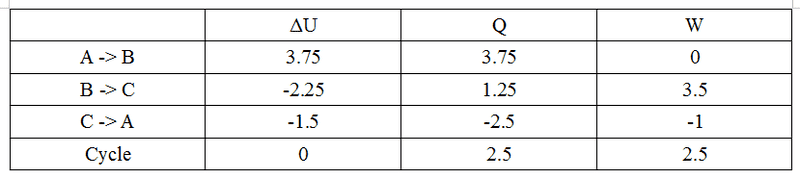But I got this problem incorrect. What errors have I made?

Last edited:
Your work looks correct. Are you sure the work asked is the work done by the gas?

ehild

But I got this problem incorrect. What errors have I made?
Your method is correct but you have to check your units. In the MKS system the units for pressure are Pascals and the units for volume are m3. Your PV diagram is in atmospheres and litres.

So, for example, the work done by the gas from B to C is not 3.5 Joules but 3.5 atm. litres = 3.5(101325Pa) 10-3 m3 =350 J.

AM

AM is right. You used litres and atm as units, then you got also the energy, heat, and and work in units (Latm) instead of joules.

ehild